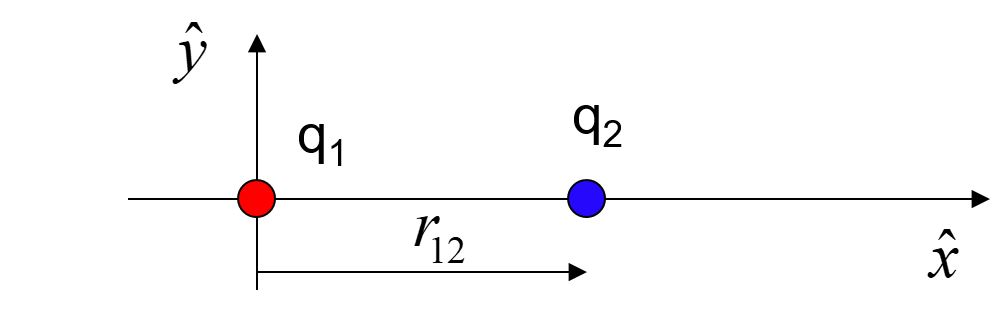﻿ Electromagnetic force

# Electric force

## Why is the Earth spherical, while an astronaut is not?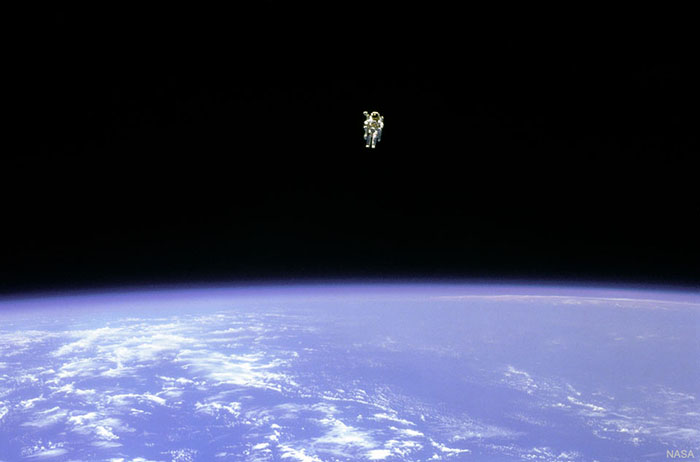The gravitational force is much weaker than the electromagnetic force. Most objects made out of normal matter have both positive and negative charge, so much of the effect of the electromagnet force is not noticeable on a large scale. The charges cancel out. Massive particles all attract each other. For a very massive body like Earth, the gravitational force dominates the electromagnetic force overall.The gravitational force is an inverse square function. As you double the distance between massive particles, the force between them decreases by a factor of four.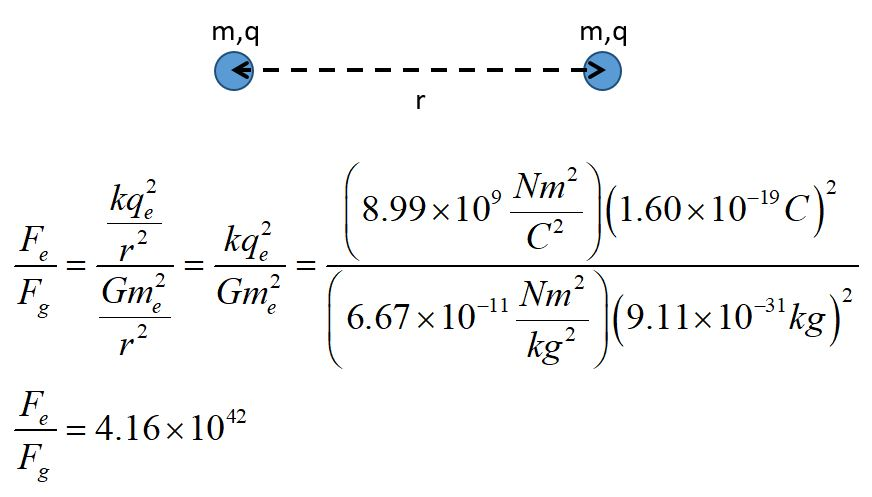The electromagnetic force is also an inverse square function the comparison of the electromagnetic force and the gravitational force between two electrons reveals the tremendous difference between the strengths of these forces.The force between two charges is described by Coulomb's law. Notice that this is a scalar equation as written above. This form just gives the magnitude of the force. The direction of the force must be determined by the signs of the charges. We typically use the variable "q" for the charge. Notice that the charge of an electron in the equation above is given in units of coulombs.

# Sample problems

## 1. Assume these five charges are equally spaced along a straight line. The red charges are positive and the blue charge is negative. Which direction will the charge in the middle accelerate?## 3. Four charges lie in a plane, at the corners of a square as shown. In which direction will the positive (red) charge accelerate?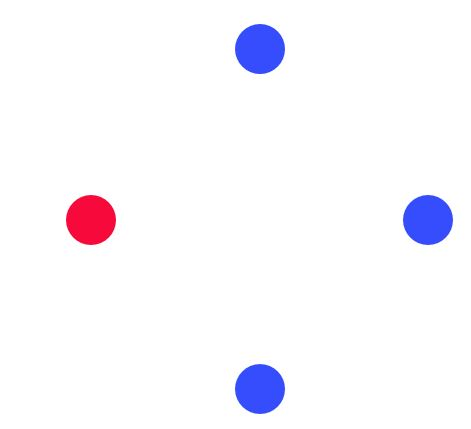## 4. Suppose q1 = -3.0 nC, q2 = 2.0 nC and q3 = -4.0 nC. Find the magnitude and direction of the total force on q2.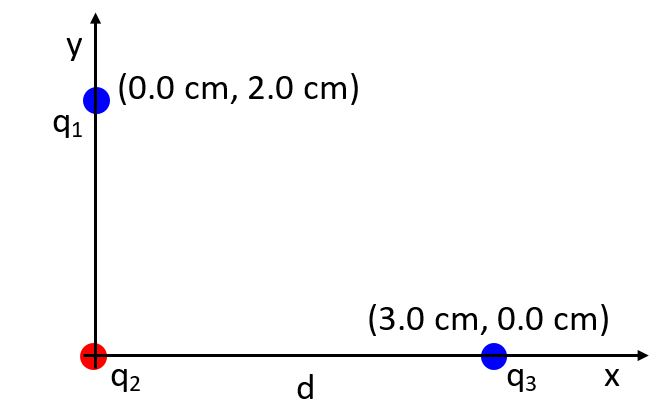## 5. Consider the charges as shown, with q2 = 3q1. Where could you place a third charge, so that it would not accelerate?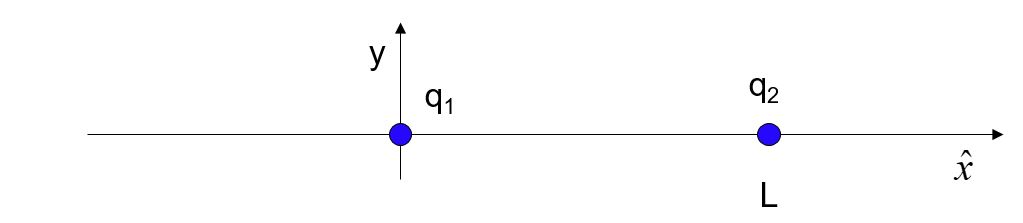## 7. Consider the charges as shown, with q2 = -3q1. Where could you place a third charge, such that the system would be in static equilibrium?## 9. Consider two charges as shown, 2.00 cm apart. Assume q1 = 3.00 nC and q2 = -1.00 nC. Find the magnitude, sign and position of a third charge, q3, that would make the system in static equilibrium.